# 高速电气化铁路电磁环境问题Review of Electromagnetic Environment in High-Speed Electrified Railways

• 全文下载: PDF(1013KB)    PP.371-379   DOI: 10.12677/JEE.2018.64044
• 下载量: 361  浏览量: 1,025

High-speed electrified railways have a great development in China, and it poses great negative effect on electromagnetic environment along electrified railways including low frequency interference and high frequency interference. In order to research the effect of low frequency interference, current distributions of autotransformer (AT) power supply type are accurately calculated, which is adopted in high-speed electrified railways. Inductance coupling and capacitance coupling are extremely small because of AT type having many wonderful features, and resistance coupling is needed to be calculated only when short-circuit fault happens. In order to research the effect of high frequency interference, the electric sparks and the passive scattering interference are analyzed thoroughly in this paper. The switch electric sparks model is in detail analyzed to be used to present approximately the electric sparks. The electric sparks are very complicated, and the precise description still remains to be further studied in a numerically exact way. The general analytical methods are given to calculate the passive scattering interference which is attributable to the architectures of high-speed electrified railways. These conclusions obtained in this paper about low frequency interference and high frequency interference can supply a foundation for further researches of electromagnetic environment along high-speed electrified railways.

1. 引言

1) 我国人口密度在世界上属于高位，各种用电设施和高速铁路接近的情况更加严重；

2) 近些年来，我国经济发展迅速，给高速铁路的设计和建设提出了更高的要求。

2. 高速铁路的供电方式

2.1. 电气化铁路供电方式Figure 1. Interferences of high-speed electrified railwaysTable 1. System resulting data of standard experiment

2.2. AT供电方式

AT供电方式，发展于上世纪70年代，因其能有效减轻牵引网对通信线路的干扰，又能适应高速、重载的优点，在我国高速铁路的建设中均推荐采用这种供电方式。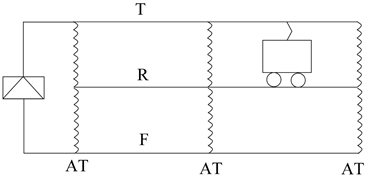Figure 2. AT power supply type

2.3. AT网络电流分布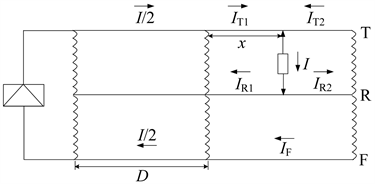Figure 3. Current distributions of AT type

${I}_{\text{T}1}=\left(1-\frac{x}{2D}\right)\cdot I$ (1)

${I}_{\text{T}2}=\frac{x}{2D}\cdot I$ (2)

${I}_{\text{F}}=\frac{x}{2D}\cdot I$ (3)

${I}_{\text{R1}}=\left(1-\frac{x}{D}\right)\cdot I$ (4)

${I}_{\text{R2}}=\frac{x}{D}\cdot I$ (5)

${I}_{\text{T}1}=\frac{3}{4}I$ (6)

${I}_{\text{T}2}=\frac{1}{4}I$ (7)

${I}_{\text{F}}=\frac{1}{4}I$ (8)

${I}_{\text{R}1}=\frac{1}{2}I$ (9)

${I}_{\text{R}2}=\frac{1}{2}I$ (10)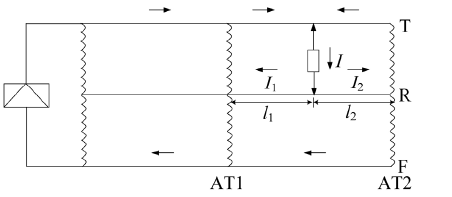Figure 4. Interference analysis of AT type

${I}_{1}=\frac{{l}_{2}}{{l}_{1}+{l}_{2}}\cdot I$ (11)

${I}_{2}=\frac{{l}_{1}}{{l}_{1}+{l}_{2}}\cdot I$ (12)

${I}_{1}\cdot {l}_{1}=\frac{{l}_{2}{l}_{1}}{{l}_{1}+{l}_{2}}\cdot I$ (13)

${I}_{2}\cdot {l}_{2}=\frac{{l}_{1}{l}_{2}}{{l}_{1}+{l}_{2}}\cdot I$ (14)

AT方式在电磁影响方面表现十分优越，其主要原因是：正馈线的回流能抵消大部分接触网电流产生的磁影响；正馈线电位与接触网电位大小接近，极性相反，从而减小了电影响。AT方式的钢轨电流，特别是由钢轨漏泄入地的漏泄电流大幅度减小，且供电回路的对称性较好，对周围环境的电磁影响大幅度减小。

3. 低频干扰

3.1. 感性耦合

3.2. 容性耦合

3.3. 阻性耦合

${u}_{M}\approx \frac{\rho {\gamma }_{p}{I}_{d}\lambda }{2\text{π}}\left[v+0.116-\mathrm{ln}v\right]$ (15)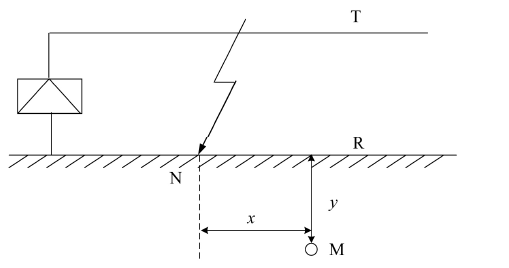Figure 5. Resistance coupling

3.4. 谐波干扰

4. 高频干扰

4.1. 弓网电火花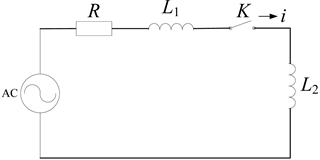Figure 6. Equivalent circuit model of traction power supply system

R是牵引回路总电阻(W)；L1是地面牵引变压器的等效电感、接触网导线电感、钢轨电感之和(H)；L2是车载牵引变压器的等效电感(H)；用开关K的断开，来等效弓网分离的状态。当回路有电流i时，断开开关K，则电流将由i变为0。如果变化过程时间 $t\to 0$ ，那么开关电压将趋于无穷大：

$\left({L}_{1}+{L}_{2}\right)\frac{\text{d}i}{\text{d}t}\to \infty$ (16)

4.2. 无源散射

5. 高速铁路电磁环境的相关标准

5.1. 低频电磁场和高频电磁场的生物效应

5.2. 有关环境噪声和电磁辐射的标准

6. 结论

1) 对低频干扰，由于高速铁路采用AT方式供电和新型的“交–直–交”电力机车，使得感性耦合、容性耦合和谐波干扰极小；而阻性耦合也仅在发生短路故障情况下，才需要考虑。

2) 对高频干扰，弓网电火花已经成为制约我国高速铁路发展的技术瓶颈。叙述了弓网电火花的研究现状，弓网电火花的危害和开关模型，为深入开展弓网电火花的研究打下一定基础。

3) 对高频干扰，无源散射，目前在国内外还没有见到相关的研究报道。对此文中给出了一般的分析思路和处理方法，为下一步的深入研究打下一定基础。

  马其祥. 强电线路的电磁影响与防护[M]. 北京: 中国铁道出版社, 1989.  谭秀炳. 交流电气化铁道牵引供电系统[M]. 成都: 西南交通大学出版社, 2007.  王洪帅, 徐青山, 袁宇波. 电气化铁路AT牵引供电方式电流分布的理论计算及仿真[J]. 江苏电机工程, 2011, 30(4): 34-38.  Machczynski, W. (1982) Currents and Potentials in Earth-Return Circuits Exposed to Alternating Current Electric Railways. IEE Proceedings B Electric Power Applications, 129, 279-288. https://doi.org/10.1049/ip-b.1982.0040  Mariscotti, A. (2003) Distribution of the Traction Return Current in AC and DC Electric Railway Systems. IEEE Transactions on Power Delivery, 18, 1422-1432. https://doi.org/10.1109/TPWRD.2003.817786  Gao, Y.G. and Li, L. (2000) An Analysis of Magnetic Field Environment near High-Voltage Power Lines and Contact Wires of Electric Railways. The Journal of China Universities of Posts and Telecommunications, 7, 78-81.  马双武, 高攸纲. 强电线交叉跨越时对通信线的磁耦合[J]. 北京邮电大学学报, 2000, 23(1): 21-25.  Mariscotti, A. and Pozzobon, P. (2000) Measurement of the Resistance and Internal Inductance of Traction Rails at 50 Hz. IEEE Transactions on Instrumentation and Measurement, 49, 294-299. https://doi.org/10.1109/19.843067  王邠, 王泉啸. 高速铁路牵引网感应电压的研究[J]. 铁道工程学报, 2011, 150(3): 77-80.  Nedelchev, N. (1997) Influence of Earth Connection on the Operation of Railway Track Circuits. IEEE Transactions on Power Delivery, 14, 215-219.  Menter, F.E. and Grcev, L.D. (1994) EMTP-Based Model for Grounding System Analysis. IEEE Transactions on Power Delivery, 9, 1838-1849. https://doi.org/10.1109/61.329517  Mellitt, B., Allan, J., Shao, Z.Y., et al. (1990) Computer-Based Methods for Induced-Voltage Calculations in AC Railways. IEE Proceedings B (Electric Power Applications), 137, 59-72. https://doi.org/10.1049/ip-b.1990.0006  高宗宝, 吴广宁, 吕玮, 等. 高速电气化铁路中的弓网电弧现象研究综述[J]. 高压电器, 2009, 45(3): 104-108.  Cozza, A. and Demoulin, B. (2008) On the Modeling of Electric Railway Lines for the Assessment of Infrastructure Impact in Radiated Emission Tests of Rolling Stock. IEEE Transactions on Electromagnetic Compatibility, 50, 566-576. https://doi.org/10.1109/TEMC.2008.924387  Ala, G. and Silvestre, M.L.D. (2002) A Simulation Model for Electromagnetic Transients in Lightning Protection Systems. IEEE Transactions on Electromagnetic Compatibility, 44, 539-554. https://doi.org/10.1109/TEMC.2002.804773  Grcev, L.D. and Dawalibi, F. (1990) An Electromagnetic Model for Transients in Grounding Systems. IEEE Transactions on Power Delivery, 5, 1773-1781. https://doi.org/10.1109/61.103673  Kubo, S. and Kato, K. (1999) Effect of Arc Discharge on the Wear Rate and Wear Mode Transition of a Copper-Impregnated Metalized Carbon Contact Strip Sliding Against a Copper Disk. Tribology International, 32, 367-378. https://doi.org/10.1016/S0301-679X(99)00062-6  陈嵩, 沙斐, 王国栋. 电气化铁道脉冲电磁骚扰的Simulink模型[J]. 铁道学报, 2009, 31(1): 55-58.  刘国福. 对我国发展高速电气化铁路接触网的思考和探讨[J]. 铁道工程学报, 2003, 77(1): 122-125.  朱绍忠, 朱连标, 王起恩, 等. 电气化铁路工频电磁场对作业工人健康的影响[J]. 环境与职业医学, 2002, 19(2): 97-99.  贺峰, 彭世蕤. 电气化铁路电磁干扰对情报雷达的影响分析[J]. 现代雷达, 2005, 27(1): 12-15.  王邠. 交流电气化铁道通信电磁防护的研究[J]. 铁道工程学报, 2007, 102(3): 199-202.  杨斌, 李明茂. 适用于高速电气化铁路的铜合金接触线[J]. 铁道机车车辆, 2005, 25(1): 68-70.  尹坚. 电气化铁路及城市轨道交通建设项目电磁环境影响评价标准探讨[J]. 铁道标准设计, 2011(8): 101-104.  包家立. 极低频电磁场的健康效应[J]. 高电压技术, 2015, 41(8):2550-2561.  李刚, 杨英超, 林凌, 等. 电磁场的生物效应的研究现状与展望[J]. 生命科学仪器, 2008, 6(3): 3-7.